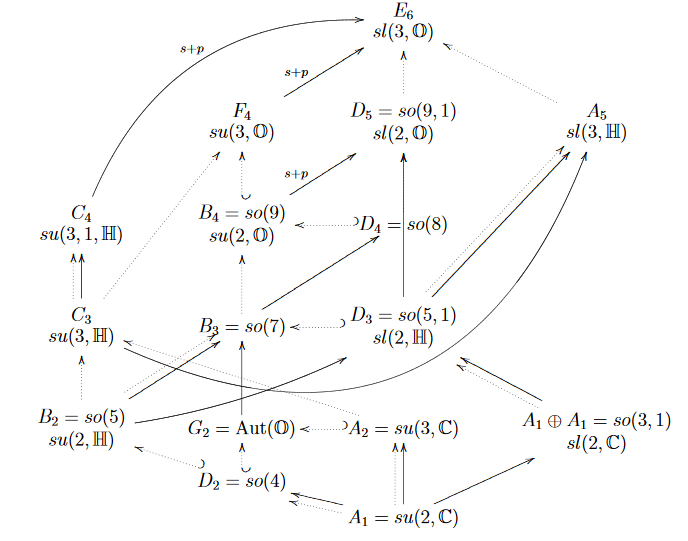group theory

Contents

Idea

One of the exceptional Lie groups.

Properties

As automorphisms of the exceptional Jordan algebra

The group of determinant-preserving linear isomorphisms of the vector space underlying the octonionic Albert algebra is ${E}_{6(-26)}$. (see e.g. (Manogue-Dray 09)).

This may be written as $SL(3,\mathbb{O})$. (Dray-Manogue 09a (16))graphics grabbed from (Dray-Manogue 09a, p. 12)

Outer automorphism

The Dynkin diagram of $E_6$ has 2-fold symmetry, corresponding to a nontrivial outer automorphism of order 2. $E_6$ acts on the octonionic projective plane and its outer automorphism gives rise to a projective duality in its geometry.

As part of the ADE pattern

Dynkin diagram/
Dynkin quiver
dihedron,
Platonic solid
finite subgroups of SO(3)finite subgroups of SU(2)simple Lie group
$A_{n \geq 1}$cyclic group
$\mathbb{Z}_{n+1}$
cyclic group
$\mathbb{Z}_{n+1}$
special unitary group
$SU(n+1)$
A1cyclic group of order 2
$\mathbb{Z}_2$
cyclic group of order 2
$\mathbb{Z}_2$
SU(2)
A2cyclic group of order 3
$\mathbb{Z}_3$
cyclic group of order 3
$\mathbb{Z}_3$
SU(3)
A3
=
D3
cyclic group of order 4
$\mathbb{Z}_4$
cyclic group of order 4
$2 D_2 \simeq \mathbb{Z}_4$
SU(4)
$\simeq$
Spin(6)
D4dihedron on
bigon
Klein four-group
$D_4 \simeq \mathbb{Z}_2 \times \mathbb{Z}_2$
quaternion group
$2 D_4 \simeq$ Q8
SO(8), Spin(8)
D5dihedron on
triangle
dihedral group of order 6
$D_6$
binary dihedral group of order 12
$2 D_6$
SO(10), Spin(10)
D6dihedron on
square
dihedral group of order 8
$D_8$
binary dihedral group of order 16
$2 D_{8}$
SO(12), Spin(12)
$D_{n \geq 4}$dihedron,
hosohedron
dihedral group
$D_{2(n-2)}$
binary dihedral group
$2 D_{2(n-2)}$
special orthogonal group, spin group
$SO(2n)$, $Spin(2n)$
$E_6$tetrahedrontetrahedral group
$T$
binary tetrahedral group
$2T$
E6
$E_7$cube,
octahedron
octahedral group
$O$
binary octahedral group
$2O$
E7
$E_8$dodecahedron,
icosahedron
icosahedral group
$I$
binary icosahedral group
$2I$
E8

As U-duality group of 5d SuGra

$E_6$ is the U-duality group (see there) of 11-dimensional supergravity compactified to 5 dimensions.

supergravity gauge group (split real form)T-duality group (via toroidal KK-compactification)U-dualitymaximal gauged supergravity
$SL(2,\mathbb{R})$1$SL(2,\mathbb{Z})$ S-duality10d type IIB supergravity
SL$(2,\mathbb{R}) \times$ O(1,1)$\mathbb{Z}_2$$SL(2,\mathbb{Z}) \times \mathbb{Z}_2$9d supergravity
SU(3)$\times$ SU(2)SL$(3,\mathbb{R}) \times SL(2,\mathbb{R})$$O(2,2;\mathbb{Z})$$SL(3,\mathbb{Z})\times SL(2,\mathbb{Z})$8d supergravity
SU(5)$SL(5,\mathbb{R})$$O(3,3;\mathbb{Z})$$SL(5,\mathbb{Z})$7d supergravity
Spin(10)$Spin(5,5)$$O(4,4;\mathbb{Z})$$O(5,5,\mathbb{Z})$6d supergravity
E6$E_{6(6)}$$O(5,5;\mathbb{Z})$$E_{6(6)}(\mathbb{Z})$5d supergravity
E7$E_{7(7)}$$O(6,6;\mathbb{Z})$$E_{7(7)}(\mathbb{Z})$4d supergravity
E8$E_{8(8)}$$O(7,7;\mathbb{Z})$$E_{8(8)}(\mathbb{Z})$3d supergravity
E9$E_{9(9)}$$O(8,8;\mathbb{Z})$$E_{9(9)}(\mathbb{Z})$2d supergravityE8-equivariant elliptic cohomology
E10$E_{10(10)}$$O(9,9;\mathbb{Z})$$E_{10(10)}(\mathbb{Z})$
E11$E_{11(11)}$$O(10,10;\mathbb{Z})$$E_{11(11)}(\mathbb{Z})$
• G2, F4,

E6, E7, E8, E9, E10, E11, $\cdots$

A description of $E_{6(-26)}$ as the special linear group in dimension 3 with values in the octonions is given in

• Corinne Manogue, Tevian Dray, Octonions and the Structure of $E_6$ Comment. Math. Univ. Carolin., 51:193–207, 2010.

• Aaron Wangberg, Tevian Dray, $E_6$, the Group: The structure of $SL(3,\mathbb{O})$ (arXiv:1212.3182)

Cohomological properties are discussed in

• Mamoru Mimura, Yuriko Sambe, Michishige Tezuka, Cohomology mod 3 of the classifying space of the exceptional Lie group $E_6$, I : structure of Cotor (arXiv:1112.5811),

Cohomology mod 3 of the classifying space of the exceptional Lie group $E_6$, II : The Weyl group invariants (arXiv:1201.3414)